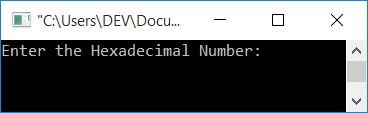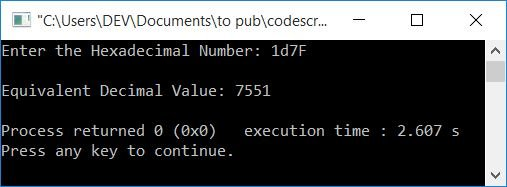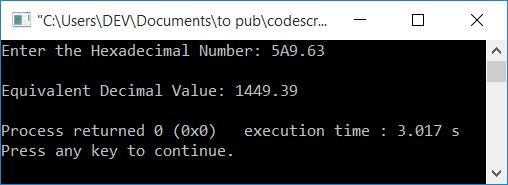# C++ Program to Convert Hexadecimal to Decimal

In this article, you will learn and get code on hexadecimal to decimal conversion in C++. Here is the list of programs you will go through:

Before going through these programs, if you're not aware of some simple steps and the formula used for the conversion, you can refer to Hexadecimal to Decimal to get all the required information.

## Hexadecimal to Decimal in C++

To convert a number from hexadecimal to decimal in C++ programming, you have to ask the user to enter the hexadecimal number first. and then convert it into its equivalent decimal value. as shown in the program given below.

The question is: write a program in C++ that converts a hexadecimal number to a decimal number. Here is its answer:

```#include<iostream>
#include<math.h>
using namespace std;
int main()
{
int decimalNum=0, rem, i=0, len=0;
char hexDecNum;
cin>>hexDecNum;
while(hexDecNum[i]!='\0')
{
len++;
i++;
}
len--;
i=0;
while(len>=0)
{
rem = hexDecNum[len];
if(rem>=48 && rem<=57)
rem = rem-48;
else if(rem>=65 && rem<=70)
rem = rem-55;
else if(rem>=97 && rem<=102)
rem = rem-87;
else
{
cout<<"\nInvalid Hex Digit!";
cout<<endl;
return 0;
}
decimalNum = decimalNum + (rem*pow(16, i));
len--;
i++;
}
cout<<"\nEquivalent Decimal Value: "<<decimalNum;
cout<<endl;
return 0;
}```

This program was built and runs under the Code::Blocks IDE. Here is its sample run:Now supply any hexadecimal number input, say 1D7F, and press the ENTER key to convert. This prints its equivalent decimal value as shown in the snapshot given below:The program doesn't care about the case of the alphabet A-F (hex characters correspond to hex digits 10 to 15). That is, as shown in the output below, either entered in lowercase or uppercase:The dry run of the above program with hexadecimal input 1D7F goes like this:

• Initial values are as follows: decimalNum=0, i=0, len=0
• When the user enters a hexadecimal number, say 1D7F, then it gets stored in hexDecNum in a way that:
• hexDecNum=1
• hexDecNum=D
• hexDecNum=7
• hexDecNum=F
• and a null terminated character \0 gets assigned to the fourth index. That is, hexDecNum=\0.
• The length of hexDecNum[] is now calculated using the while loop. That is, how many characters were entered as hexadecimal input? The while loop evaluates in this way:
• The condition hexDecNum[i]!='\0' or hexDecNum!='\0' or 1!='\0' evaluates to be true, therefore program flow goes inside the loop, and increments the value of both variables, say len and i. So, i=1 and len=1.
• The program flow goes back and evaluates the condition again. That is, the condition hexDecNum[i]!='\0' or hexDecNum!='\0' or D!='\0' evaluates to be true again, therefore the program flow again goes inside the loop and increments the value of both variables.
• The while loop's evaluation continues until the condition is determined to be false. That is, when the value of i equals 4. The condition evaluates to false because there is no character available at that index (the fourth index). Therefore, it gets matched with a null terminated character (\0).
• Now the value of len gets decremented. As a result, len = 3 and i = 0.
• The condition of the second while loop gets evaluated.
• That is, the condition len>=0 or 3>=0 evaluates to be true, therefore program flow goes inside the loop.
• There, hexDecNum[len], hexDecNum, or F is set to rem.
• Because rem is of the int (integer) type, the ASCII value of F gets initialized to rem. So rem=70
• Now, using if-else, we've compared the value of rem (with ASCII values of 0-9, A-F and a-f) and processed the statement accordingly.
• That is, because rem (70) is neither greater than or equal to 48 nor less than or equal to 57. Therefore, the first else if's condition gets evaluated. That is, the condition rem<=70 or 70<=70 evaluates to be true, therefore rem-55 or 70-55 or 15 gets initialized to rem. 15 corresponds to F.
• decimalNum + (rem*pow(16, i)) or 0 + (15*pow(16, 0)) or 15*160 or 15*1 or 15 is set to decimalNum.
• The value of len gets decremented. So len=2
• and the value of i gets incremented. So i=1
• Now program flow goes back, and the condition of the while loop gets evaluated.
• That is, the condition len>=0 or 2>=0 again evaluates to be true, so the program flow again goes inside the loop, and it processes the code in a similar way as told above.
• Continue the process in a similar way until the condition evaluated as false.
• If we continue the process, we will obtain the values as follows:
• rem=70, rem=15, decimalNum=15, len=2, i=1
• rem=55, rem=7, decimalNum=127, len=1, i=2
• rem=68, rem=13, decimalNum=3455, len=0, i=3
• rem49, rem=1, decimalNum=7551, len=(-1), i=4)
• Because the value of len is now less than 0, the condition evaluates to be false, and the evaluation of the while loop gets ended.
• As output, print the value of decimalNum. That is, 7551

You can also compare and process the hexDecNum[] characters one by one. To do this, replace the following block of code:

```if(rem>=48 && rem<=57)
rem = rem-48;
else if(rem>=65 && rem<=70)
rem = rem-55;
else if(rem>=97 && rem<=102)```

with the block of code given below:

```if(rem>='0' && rem<='9')
rem = rem-48;
else if(rem>='A' && rem<='F')
rem = rem-55;
else if(rem>='a' && rem<='f')```

### Hexadecimal to decimal in C++ without using the pow() function

If you don't want to use the pow() function in the math.h header file, then you can replace the following statement:

`decimalNum = decimalNum + (rem*pow(16, i));`

with

```k=1;
mul=1;
while(k<=i)
{
mul=16*mul;
k++;
}
decimalNum = decimalNum + (rem*mul);```

Note: Don't forget to declare both new variables k and mul at the start of the program. And remove the header file, math.h. Everything else will remain the same.

### What if a hexadecimal number contains fractional parts?

To deal with a type of input that contains fractional parts. If it is a hexadecimal number containing a fractional part (or decimal point), use the following program:

```#include<iostream>
#include<math.h>
using namespace std;
int main()
{
int decimalNum=0, decNumOne=0, dotPosition=0;
int rem, i=0, len=0, lenTemp;
float decNumTwo=0;
char hexDecNum;
cin>>hexDecNum;
while(hexDecNum[i]!='\0')
{
if(hexDecNum[i]=='.')
dotPosition = i;
len++;
i++;
}
len--;
i=0;
if(dotPosition==0)
{
while(len>=0)
{
rem = hexDecNum[len];
if(rem>=48 && rem<=57)
rem = rem-48;
else if(rem>=65 && rem<=70)
rem = rem-55;
else if(rem>=97 && rem<=102)
rem = rem-87;
else
{
cout<<"\nInvalid Hex Digit!";
cout<<endl;
return 0;
}
decimalNum = decimalNum + (rem*pow(16, i));
len--;
i++;
}
cout<<"\nEquivalent Decimal Value: "<<decimalNum;
}
else
{
lenTemp = dotPosition-1;
while(lenTemp>=0)
{
rem = hexDecNum[lenTemp];
if(rem>=48 && rem<=57)
rem = rem-48;
else if(rem>=65 && rem<=70)
rem = rem-55;
else if(rem>=97 && rem<=102)
rem = rem-87;
else
{
cout<<"\nInvalid Hex Digit!";
cout<<endl;
return 0;
}
decNumOne = decNumOne + (rem*pow(16, i));
lenTemp--;
i++;
}
lenTemp = dotPosition+1;
i=-1;
while(lenTemp<=len)
{
rem = hexDecNum[lenTemp];
if(rem>=48 && rem<=57)
rem = rem-48;
else if(rem>=65 && rem<=70)
rem = rem-55;
else if(rem>=97 && rem<=102)
rem = rem-87;
else
{
cout<<"\nInvalid Hex Digit!";
cout<<endl;
return 0;
}
decNumTwo = decNumTwo + (rem*pow(16, i));
lenTemp++;
i--;
}
decNumTwo = decNumOne+decNumTwo;
cout<<"\nEquivalent Decimal Value: "<<decNumTwo;
}
cout<<endl;
return 0;
}```

Here is its sample run with the hexadecimal number input as 5A9.63.The variable dotPosition is used here to check whether decimal points are available in the hexadecimal input or not. If hexadecimal input contains a fractional part (or has a decimal point), then 1 gets initialized to the dotPosition variable. Later, it is checked to see if its value is equal to 0 or not.If it is equal to 0 (meaning 1 does not get initialized to it), then process the normal conversion; otherwise, deal with different rules as shown in the above program.

That is, if a hexadecimal number contains a fractional part (or decimal point), then we've used two parts. One before and one after the decimal point.

The part that is available before decimal gets converted into its equivalent decimal value and initialized to decNumOne. Whereas the part after decimal is converted to its equivalent decimal value and initialized to decNumTwo (a float type variable).Finally, add and print the value.

Note: The rules for converting hexadecimal (after the decimal point) to decimal are already discussed in the article Hexadecimal (with Fractional) to Decimal.

### In C++, use Function and Pointer to convert hexadecimal to decimal

This program uses a function and pointer to convert hexadecimal to decimal in C++.

```#include<iostream>
#include<math.h>
using namespace std;
unsigned long HexDecToDec(char []);
int main()
{
unsigned long decimalNum;
char hexDecNum;
cin>>hexDecNum;
decimalNum = HexDecToDec(hexDecNum);
if(decimalNum==0)
cout<<"\nInvalid Hex Digit!";
else
cout<<"\nEquivalent Decimal Value: "<<decimalNum;
cout<<endl;
return 0;
}
unsigned long HexDecToDec(char hexDecNum[])
{
char *hexDecPointer;
int i, len = 0;
const int base = 16;
unsigned long decimalNum = 0;
// Find the len of Hexadecimal Number
for(hexDecPointer=hexDecNum; *hexDecPointer != '\0'; hexDecPointer++)
len++;
hexDecPointer = hexDecNum;
// Now convert hex digit to decimal number one by one
for(i=0; *hexDecPointer != '\0' || i<len; i++, hexDecPointer++)
{
if(*hexDecPointer>=48 && *hexDecPointer<=57)
decimalNum = decimalNum + (*hexDecPointer - 48)*pow(base, len-i-1);
else if(*hexDecPointer>=65 && *hexDecPointer<=70)
decimalNum = decimalNum + (*hexDecPointer - 55)*pow(base, len-i-1);
else if(*hexDecPointer>=97 && *hexDecPointer<=102)
decimalNum = decimalNum + (*hexDecPointer - 87)*pow(base, len-i-1);
else
len=0;
}
if(len==0)
return 0;
else
return decimalNum;
}```

Note: This program doesn't work with hexadecimal input containing fractional (decimal) parts.

To create the program using a user-defined function that converts hexadecimal (with fractional part) to its equivalent decimal value. Then you can use the program that I've already mentioned above to deal with this type of input. Simply change it and make it your own.

#### The same program in different languages

C++ Quiz

« Previous Program Next Program »